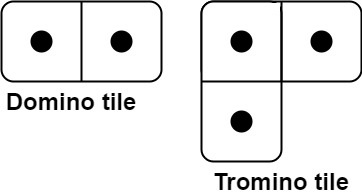790. Domino and Tromino Tiling
Medium
2.9K
886

You have two types of tiles: a `2 x 1` domino shape and a tromino shape. You may rotate these shapes.Given an integer n, return the number of ways to tile an `2 x n` board. Since the answer may be very large, return it modulo `109 + 7`.

In a tiling, every square must be covered by a tile. Two tilings are different if and only if there are two 4-directionally adjacent cells on the board such that exactly one of the tilings has both squares occupied by a tile.

Example 1:```Input: n = 3
Output: 5
Explanation: The five different ways are show above.
```

Example 2:

```Input: n = 1
Output: 1
```

Constraints:

• `1 <= n <= 1000`
Accepted
96.6K
Submissions
183.6K
Acceptance Rate
52.6%

Seen this question in a real interview before?
1/4
Yes
No

Discussion (0)

Related Topics